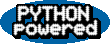File: timebothCMP.out.txt

```Output data sizes: 524288 50 50 26214400 26214400

[Python 2.6: large.txt, 2935401 bytes]

[Python 2.6: large.bin, 13168640 bytes]

[Python 2.6: testIO.out, 26214400 bytes]
write_byLines_textMode     (testIO.out=25.00M)  => 0.453313
write_byLines_binaryMode   (testIO.out=25.00M)  => 1.235918
write_byBlocks_textMode    (testIO.out=25.00M)  => 1.464019
write_byBlocks_binaryMode  (testIO.out=25.00M)  => 0.560694
write_allAtOnce_textMode   (testIO.out=25.00M)  => 0.174307
write_allAtOnce_binaryMode (testIO.out=25.00M)  => 0.579587

Output data sizes: 524288 50 50 26214400 26214400

[Python 3.0: large.txt, 2935401 bytes]

[Python 3.0: large.bin, 13168640 bytes]

[Python 3.0: testIO.out, 26214400 bytes]
write_byLines_textMode     (testIO.out=25.00M)  => 5.409266
write_byLines_binaryMode   (testIO.out=25.00M)  => 2.160819
write_byBlocks_textMode    (testIO.out=25.00M)  => 4.927914
write_byBlocks_binaryMode  (testIO.out=25.00M)  => 1.884943
write_allAtOnce_textMode   (testIO.out=25.00M)  => 1.313119
write_allAtOnce_binaryMode (testIO.out=25.00M)  => 0.603439

==Summary==

[read_byLines_textMode      (large.txt=2.80M)]   => 3.0 is   41.372 times slower
[read_byLines_binaryMode    (large.txt=2.80M)]   => 3.0 is   82.194 times slower
[read_byBlocks_textMode     (large.txt=2.80M)]   => 3.0 is   54.405 times slower
[read_byBlocks_binaryMode   (large.txt=2.80M)]   => 3.0 is    1.736 times slower
[read_allAtOnce_textMode    (large.txt=2.80M)]   => 3.0 is   62.679 times slower
[read_allAtOnce_binaryMode  (large.txt=2.80M)]   => 3.0 is  202.909 times slower
[read_byBlocks_binaryMode   (large.bin=12.56M)]  => 3.0 is    1.441 times slower
[read_allAtOnce_binaryMode  (large.bin=12.56M)]  => 3.0 is  826.735 times slower
[write_byLines_textMode     (testIO.out=25.00M)] => 3.0 is   11.933 times slower
[write_byLines_binaryMode   (testIO.out=25.00M)] => 3.0 is    1.748 times slower
[write_byBlocks_textMode    (testIO.out=25.00M)] => 3.0 is    3.366 times slower
[write_byBlocks_binaryMode  (testIO.out=25.00M)] => 3.0 is    3.362 times slower
[write_allAtOnce_textMode   (testIO.out=25.00M)] => 3.0 is    7.533 times slower
[write_allAtOnce_binaryMode (testIO.out=25.00M)] => 3.0 is    1.041 times slower
```Books Programs Blog Python Author Training Search Email ©M.Lutz